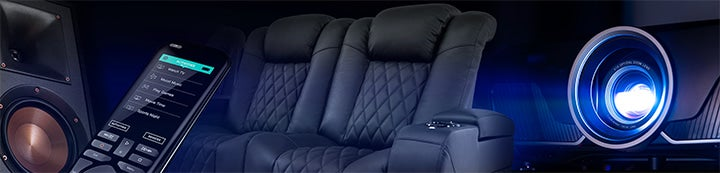Status
Not open for further replies.
1 - 7 of 7 Posts

#### Brucemck2

·
##### Registered
Joined
·
900 Posts
Discussion Starter · ·
Now have the RTA and the Behringer Ultra Pro (actually, two: one for the sub and center the other for the left and right.)

Question: how to roughly estimate the right filter size. Parameters are center frequency, gain, and width in terms of percentage of an octive.

If I have a peak that's centered (say) on 70hz, with the left and right edges of the tail at (say) 60hz and 80hz, and (say) 6db high, what would be a good starting point for the UltraPro filter.

I would assume that it should be centered on 70hz with gain of -6db, but what's an easy way to determine the proper width?

ps -- I got the sub dialed in with trial and error. Question above is meant to limit cut the number of trials.

#### JohnPM

·
##### Registered
Joined
·
617 Posts
Assuming the Ultra uses the same filter characteristic as the BFD, you could measure the width in Hz at the half gain points (e.g. if your peak is 9dB, measure at 4.5dB) and then use

octaves = 0.707*(measured bandwidth in Hz)/(centre frequency in Hz)

For example, if the width at the half gain points (3dB using your 70Hz example) were 30Hz, the width setting would be 0.707*30/70 = 0.303 octaves (or 30.3% if that is the setting offered, or 18 sixtieths of an octave for a BFD).

Regards,

#### Terry Montlick

·
##### Registered
Joined
·
3,258 Posts
Quote:
 Originally Posted by JohnPM Assuming the Ultra uses the same filter characteristic as the BFD, you could measure the width in Hz at the half gain points (e.g. if your peak is 9dB, measure at 4.5dB) and then use octaves = 0.707*(measured bandwidth in Hz)/(centre frequency in Hz) For example, if the width at the half gain points (3dB using your 70Hz example) were 30Hz, the width setting would be 0.707*30/70 = 0.303 octaves (or 30.3% if that is the setting offered, or 18 sixtieths of an octave for a BFD). Regards,
John,

Actually, the half gain points are 3 dB down. They are independent of the height of the peak.

Regards.

Terry

#### JohnPM

·
##### Registered
Joined
·
617 Posts
No, I don't mean the -3dB points, I really mean the points at which the gain in dB is half the total amount. Depends on the form of biquad used to implement the filter of course, but for the BFD (and probably the Ultra) the bandwidth is defined by the width halfway down the response shape.

#### Terry Montlick

·
##### Registered
Joined
·
3,258 Posts
Quote:
 Originally Posted by JohnPM No, I don't mean the -3dB points, I really mean the points at which the gain in dB is half the total amount. Depends on the form of biquad used to implement the filter of course, but for the BFD (and probably the Ultra) the bandwidth is defined by the width halfway down the response shape.
Interesting! I know of various definitions of bandwidth when referring to parametric equalizer biquads, but this is not one I've seen. The closest to this is Andy Moorer's use of the midpoint gain in dB, but only when the peak is less than 6 dB.

Regards,

Terry

·
Joined
·
617 Posts

#### Terry Montlick

·
##### Registered
Joined
·
3,258 Posts
Quote:
 Originally Posted by JohnPM In that case you might find this paper by RBJ interesting, reviewing the various ways of parameterising the biquad: http://www.musicdsp.org/files/EQ-Coefficients.pdf Regards,
Ah, yes. I know that paper well! I referred to it just the other day, when I needed to code a C++ parametric filter class, as a subclass of an IIR filter class I had written a while ago.

Regards,

Terry

1 - 7 of 7 Posts
Status
Not open for further replies.